# How to Add and Subtract Dates and Times in Excel?

Changing the dates on a spreadsheet can be a time-consuming process. You can change the dates without having to get out your calendar to count the days or months because you can do so directly in Microsoft Excel by using basic addition or subtraction.

Adjusting the dates does not have to be a burden, regardless of whether this is a project schedule with due dates, a budget with dates when bills are due, or an order sheet with shipment dates. You can enter the addition or subtraction, and the new dates will be automatically calculated for you after a few straightforward steps.

Suppose you want to find out what the new completion date will be for a project by adjusting the schedule date by adding some more days or weeks to it, or you want to figure out how long it will take to finish a particular activity from a list of project activities. Both of these scenarios are possible. Using a straightforward formula, you are able to modify the amount of time between two dates by adding or removing a specific number of days.

## Add or Subtract Days from a Date

Let’s see how to add or subtract days from date to see the new date through an example.

### Step 1

Suppose we have a deadline date of some project for different employees in an Excel sheet. We have to modify those deadline dates, some of them will be extended, some are already delivered before deadline date. So, we have to modify those dates and we will verify the new date. See the below given image.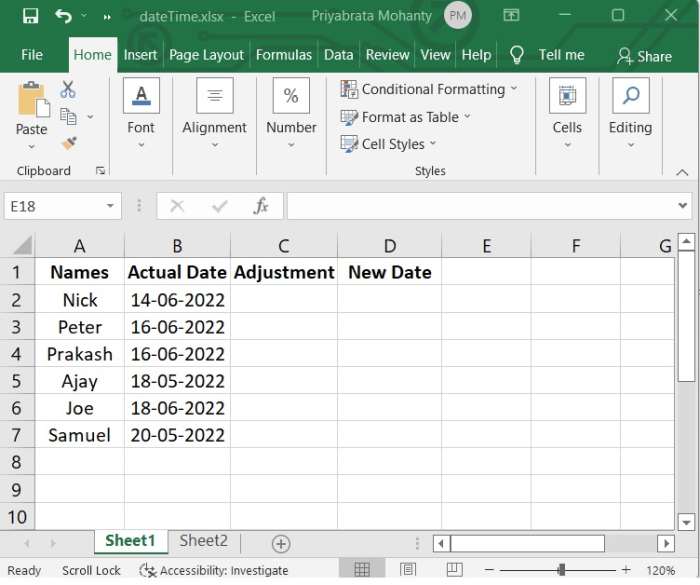Here in the "Adjustment" column, if date is extended, then the number of days will be added and if already finished, then mentioned number of days will be subtracted.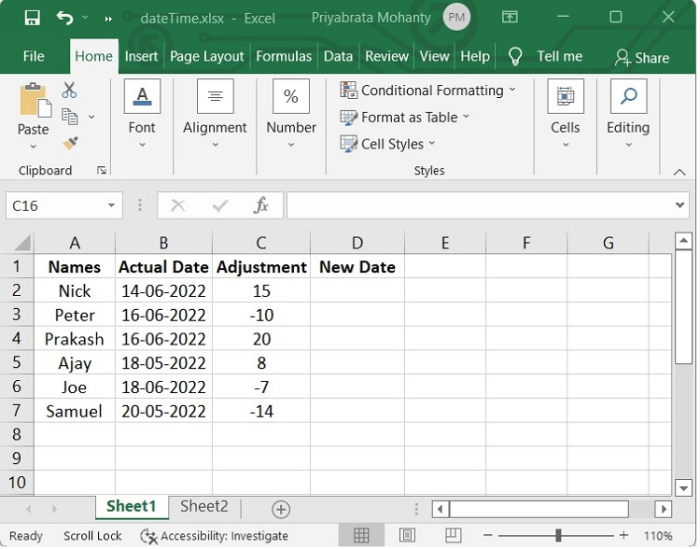### Step 2

Now we will use the following formula to create "New Date."

=(B2+C2)

See the below image to understand how it is done.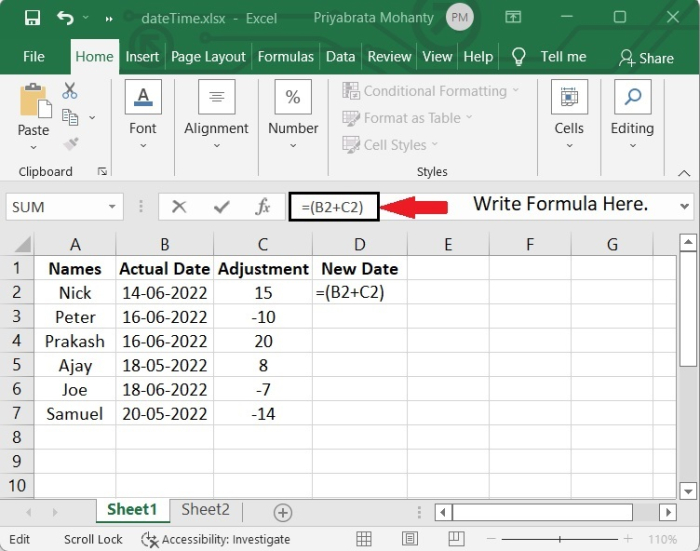After adding the formula, press Enter and we will get the New Date.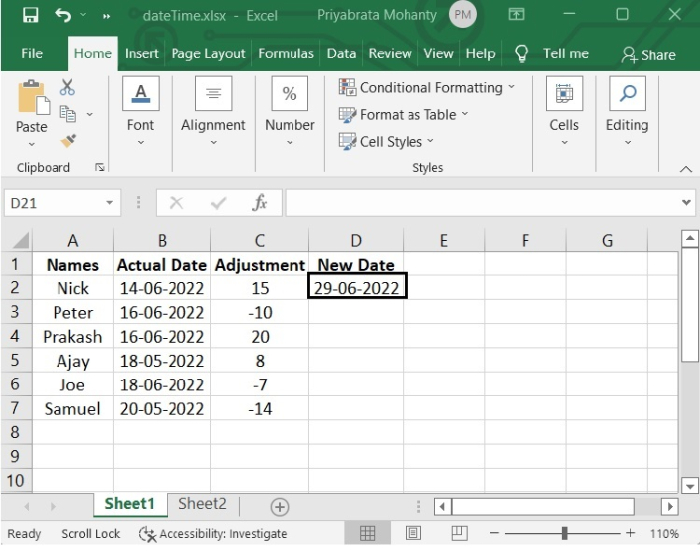### Step 3

Now to reflect in other cells, select the cell, on lower right corner of that cell, you will get a "+" symbol, which activates the autofill functionality of excel, click there and drag down to the required number of cells and you will see the reflection in each cell.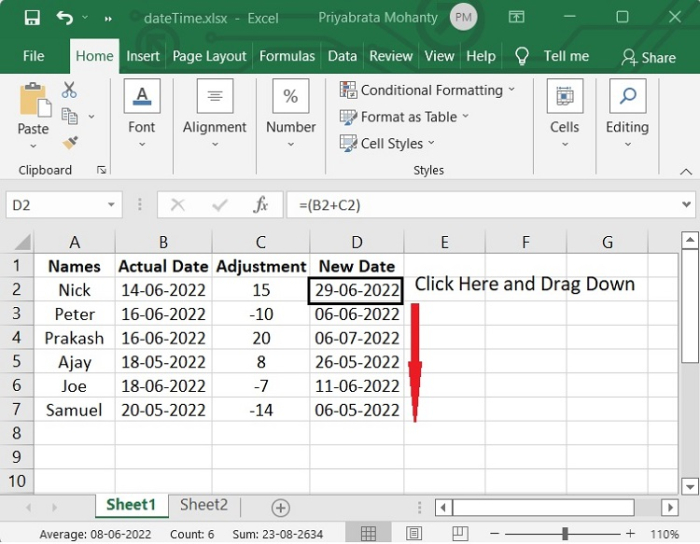## Add or Subtract Time in Excel

Consider the scenario in which you need to get at a result by adding two separate time values together. Alternately, in order to calculate the overall amount of time spent working on a project, you will need to deduct one time value from another. Let’s take an example to understand how to add and subtract time in Excel.

### Subtract Time in Excel

In our example, we have taken the start time and end time of work of some employees in a project and we will calculate the working hour of each employee.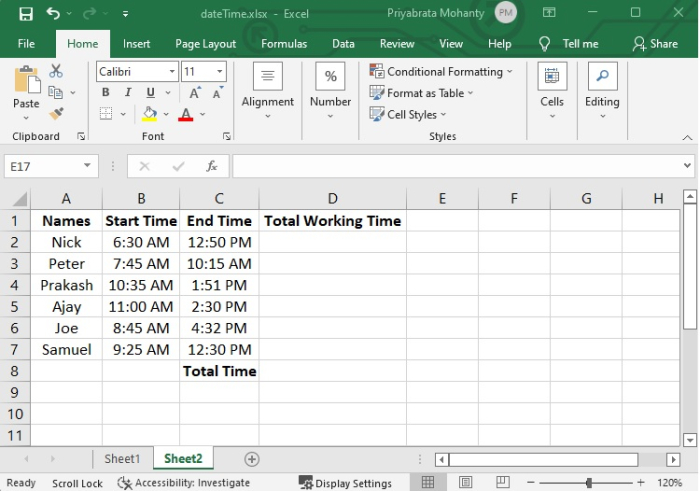### Step 1

In the example, we will use the following formula to calculate total working time of each employee.

=(C2-B2)

See the following image for reference.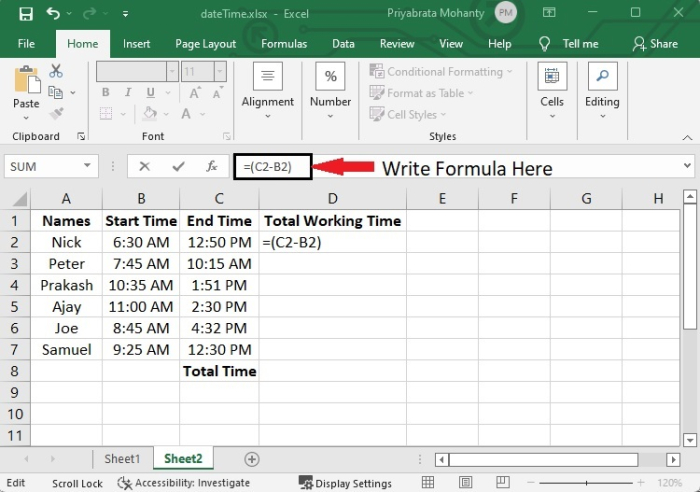After pressing Enter, you will see the total working hour of the employee.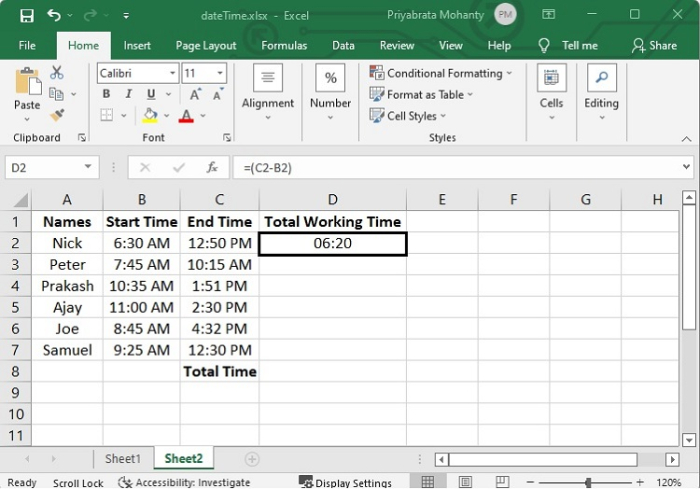### Step 2

To reflect these, select that column and drag to the required number of columns.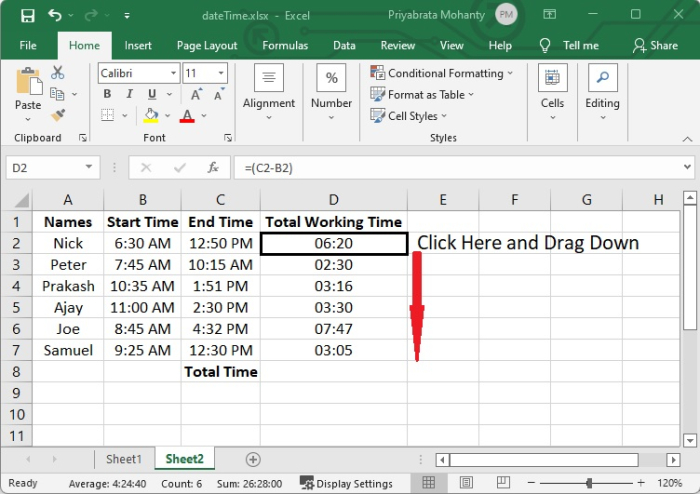Suppose there is a scenario in which we have to know the total time to complete the project. So, we have to add the working time of each employee. Let’s see how to calculate those.

To calculate total time, add all the cell data. Simply use the formula

=(D2+D3+D4+D5+D6+D7)

See the following image for reference.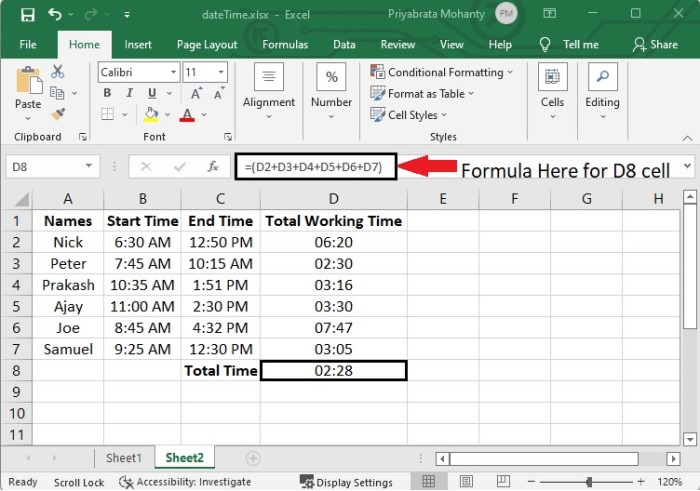After adding, you will see the time is coming 02:28. This is because the time format is in "hh:mm" format. So, to display the time that is more than 24 hours, follow the steps given below −

### Step 1

Select that column and right-click there.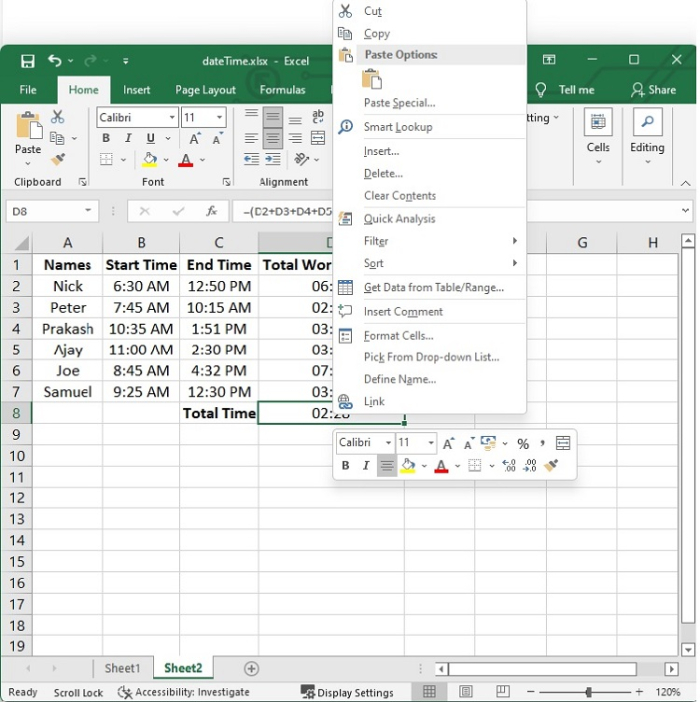### Step 2

Go to the "Format Cells" option.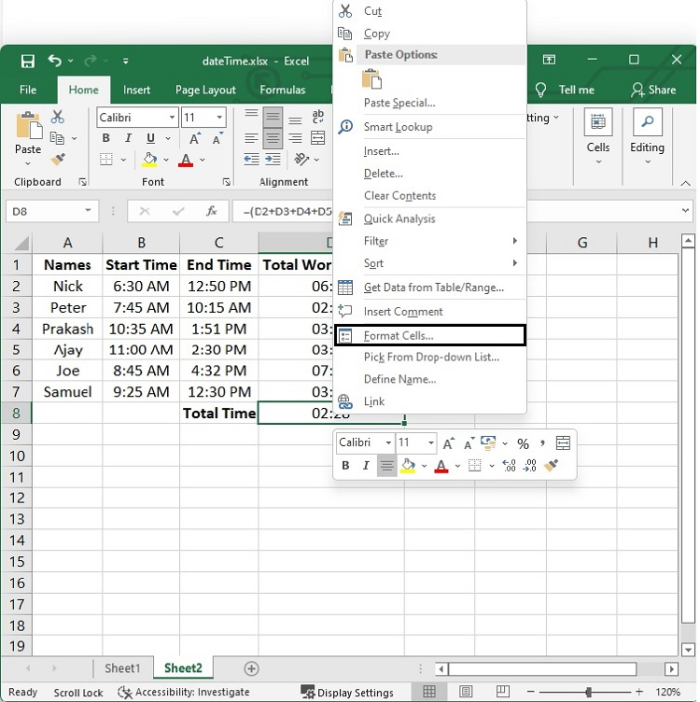### Step 3

Go to "Custom" option. In the "type" box, write [h]:mm:ss;@ and then click on OK.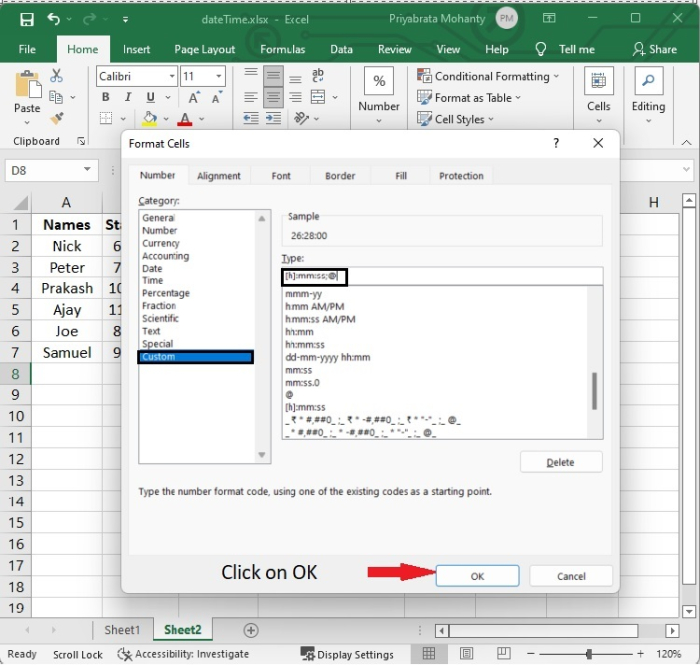Now you will see the total time is 26:28, which is more than 24 hrs. See the following image for reference.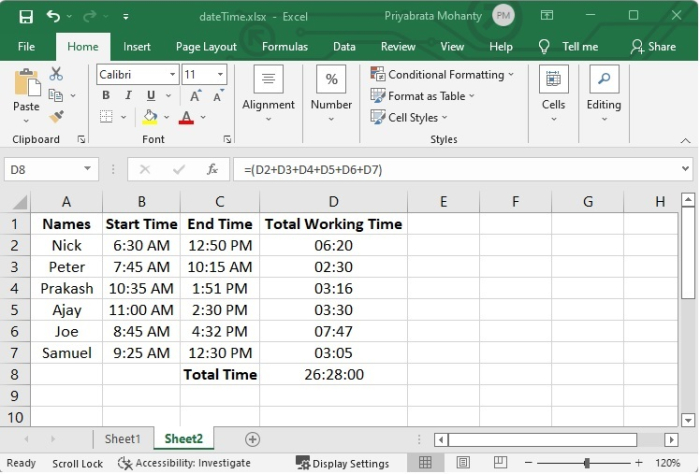## Conclusion

In this tutorial, you learnt how to add and subtract days from a specific date, and how to add and subtract, a time from another time.